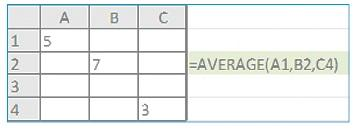# Excel formulas and functions 101

Excel really shines in its ability to perform calculations. However, it can seem somewhat intimidating if you don’t know the rules.

### The basics

All formulas begin with the equals (=) sign. And all formulas are resolved according to the Order of Operation.

• Parenthesis
• Exponents
• Multiplication
• Division
• Addition
• Subtraction

An easy way to remember it is Please, excuse my dear Aunt Sally. That means that if the formula is: =5+6*10, the answer would be 65 and not 110. In order for this formula to resolve to 110, you would need parenthesis around the 5+6 expression, so: =(5+6)*10.

When a formula doesn’t resolve the way you anticipate, try putting parenthesis around logical groups of elements. Perhaps what the formula was meant to resolve was putting a \$5 item and a \$6 item in a single package and shipping 10 of them.

Here are a few tips for working with Excel formulas:

1. Be intentional about where you click when editing a formula. Many of us perform an onscreen operation and then click down and to the right to see our handiwork. This habit will insert unwanted cell references right in the middle of your formula.
2. It may be easier to edit formulas in the cell. Press F2 to enter the edit mode in a cell. If you zoom to a larger magnification, your formula will also be magnified.
3. If you use cells from other work sheets or workbooks, you can just press Enter when you have selected your last formula cell reference. It will automatically return you to the cell in which you keyed in the formula.
4. Use arrow keys to navigate to different parts of your formula in edit mode to avoid clicking in the wrong place.

### Working with functions

Functions are like programs that do math operations. For example, you could type in the math operator “+” to add up the values 1, 5 and 6. Or, using the SUM function,  you can accomplish the same thing. With just three cells it doesn’t matter, but imagine if it were 300! SUM is easier.The syntax for all functions includes a left parenthesis after the function name and a right parenthesis after the last argument. Functions use two additional types of punctuation, commas and the colons. Commas separate “arguments.” Arguments can be thought of as parameters that the program needs to run. The names of the arguments and their arrangement, or syntax, are shown by clicking inside the parenthesis. The colon indicates a range. You can read this expression A1:A3 as from A2 through A3. We can read the formula using the SUM function in the diagram as “Create a sum with the values in the range A1 through A3.”

You can also separate nonadjacent cell references in these functions using a comma. For example, in the work sheet below, you average the values in A1, B2 and C4. To get this result with your mouse, after selecting your first value, hold your Ctrl key down while selecting the remaining cell references. This operation will insert commas automatically between cell references.